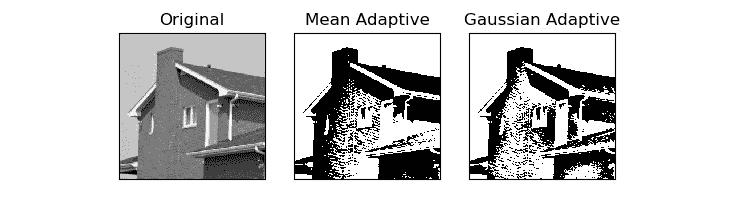• 色彩空间及其变换
• 对图像执行变换操作
• 图像的透视变换
• 对图像执行阈值

# 色彩空间及其变换

import cv2
import matplotlib.pyplot as plt
b,g,r = cv2.split (img)
img = cv2.merge((r, g, b))
plt.imshow (img)
plt.title ('COLOR IMAGE')
plt.axis('off')
plt.show()


import cv2
import matplotlib.pyplot as plt
img = cv2.cvtColor (img, cv2.COLOR_BGR2RGB)
plt.imshow (img)
plt.title ('COLOR IMAGE')
plt.axis('off')
plt.show()


import cv2
j=0
for filename in dir(cv2):
if filename.startswith('COLOR_'):
print(filename)
j = j + 1
print('There are ' + str(j) +
' Colorspace Conversion flags in OpenCV '
+ cv2.__version__ + '.')


.
.
.
.
.
COLOR_YUV420p2RGBA
COLOR_YUV420sp2BGR
COLOR_YUV420sp2BGRA
COLOR_YUV420sp2GRAY
COLOR_YUV420sp2RGB
COLOR_YUV420sp2RGBA
COLOR_mRGBA2RGBA


OpenCV 4.0.1 中有 274 个色彩空间转换标志。

## HSV 色彩空间

import cv2
import numpy as np
c = cv2.cvtColor(np.array([[[255, 0, 0]]],
dtype=np.uint8),
cv2.COLOR_BGR2HSV)
print(c)


[[[120 255 255]]]


import cv2
def emptyFunction():
pass
windowName = "Saturation Demo"
cv2.namedWindow(windowName)
cv2.createTrackbar('Saturation Level',
windowName, 0,
24, emptyFunction)
while(True):
hsv = cv2.cvtColor( img, cv2.COLOR_BGR2HSV)
h, s, v = cv2.split(hsv)
saturation = cv2.getTrackbarPos('Saturation Level', windowName)
s = s + saturation
v = v + saturation
img1 = cv2.cvtColor(cv2.merge((h, s, v)), cv2.COLOR_HSV2BGR)
cv2.imshow(windowName, img1)
if cv2.waitKey(1) == 27:
break
cv2.destroyAllWindows()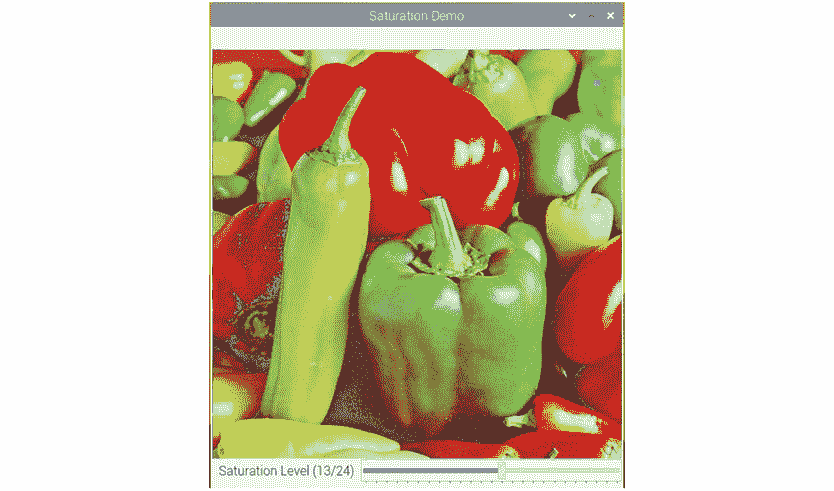## 基于颜色的实时跟踪

import numpy as np
import cv2
cap = cv2.VideoCapture(0)
while ( True ):
hsv = cv2.cvtColor(frame, cv2.COLOR_BGR2HSV)
image_mask = cv2.inRange(hsv, np.array([40, 50, 50]),
np.array([80, 255, 255]))
cv2.imshow('Original', frame)
cv2.imshow('Output', output)
if cv2.waitKey(1) == 27:
break
cv2.destroyAllWindows()
cap.release()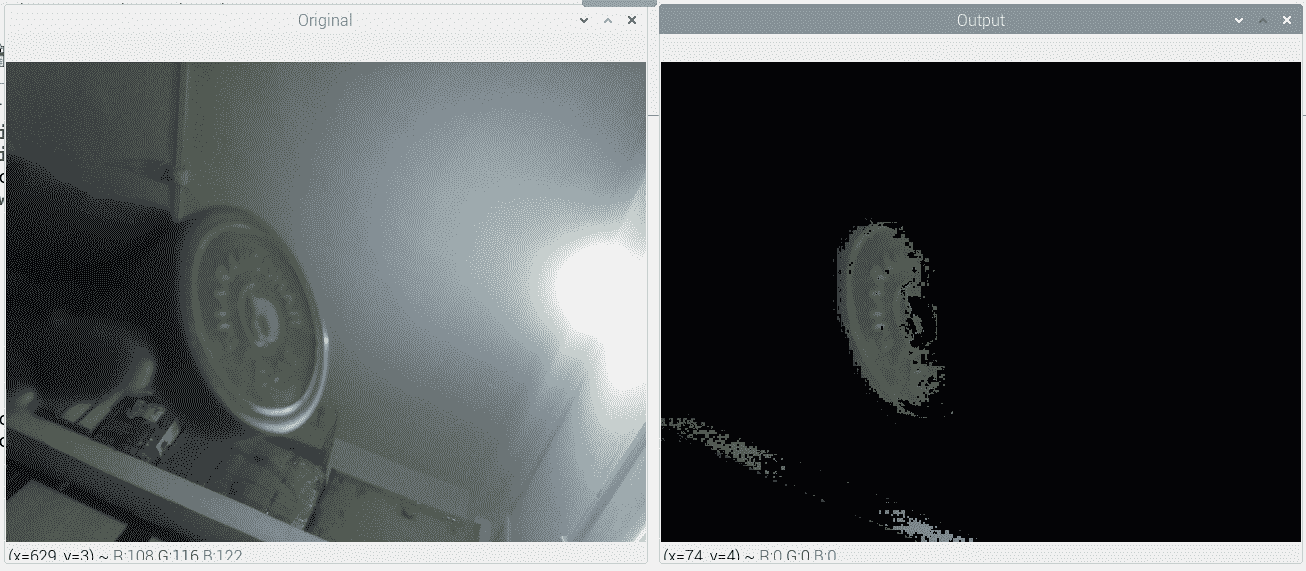cv2.imshow('Image Mask', image_mask)


blue = cv2.inRange(hsv, np.array([100, 50, 50]), np.array([140, 255, 255]))
green = cv2.inRange(hsv, np.array([40, 50, 50]), np.array([80, 255, 255]))


1. 首先，导入所有必需的库：

py import numpy as np

py import cv2

2. 然后，我们定义一个空函数：

py def emptyFunction():

py pass

3. 让我们初始化所有必需的对象和变量：

py cap = cv2.VideoCapture(0)

py windowName = 'Object Tracker'

py trackbarName = 'Color Chooser'

py cv2.namedWindow(windowName)

py cv2.createTrackbar(trackbarName,

py windowName, 0, 1,

py emptyFunction)

py color = 0

4. 在这里，我们有一个主循环：

py while (True):

py ret, frame = cap.read()

py hsv = cv2.cvtColor(frame, cv2.COLOR_BGR2HSV)

py color = cv2.getTrackbarPos(trackbarName, windowName)

py if color == 0:

py image_mask = cv2.inRange(hsv, np.array([40, 50, 50]),

py np.array([80, 255, 255]))

py else:

py image_mask = cv2.inRange(hsv, np.array([100, 50, 50]),

py np.array([140, 255, 255]))

py output = cv2.bitwise_and(frame, frame, mask=image_mask)

py cv2.imshow(windowName, output)

py if cv2.waitKey(1) == 27:

py break

5. 最后，我们摧毁了所有窗口并释放了摄像头：

py cv2.destroyAllWindows()

py cap.release()

# 对图像执行变换操作

## 缩放

• cv2.INTER_LANCZOS4：这涉及在8x8像素附近的 Lanczos 插值方法。
• cv2.INTER_CUBIC：这涉及在4x4像素邻域上的双三次插值方法，并且首选用于对图像执行缩放操作。
• cv2.INTER_AREA：这意味着使用像素面积关系进行重采样。 这对于在图像上执行缩小操作是优选的。
• cv2.INTER_NEAREST：这表示最近邻插值的方法。
• cv2.INTER_LINEAR：这表示双线性插值方法。 这是参数的默认参数。

import cv2
upscale = cv2.resize(img, None, fx=1.5, fy=1.5,
interpolation=cv2.INTER_CUBIC)
downscale = cv2.resize(img, None, fx=0.5, fy=0.5,
interpolation=cv2.INTER_AREA)
cv2.imshow('upscale', upscale)
cv2.imshow('downscale', downscale)
cv2.waitKey(0)
cv2.destroyAllWindows()


## 图像的平移，旋转和仿射变换

cv2.warpAffine()函数用于计算输入图像上的平移，旋转和仿射仿射等运算。 它接受输入图像，转换矩阵和输出图像的大小作为参数，然后返回转换后的图像。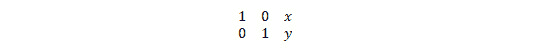import numpy as np
import cv2
import matplotlib.pyplot as plt
input=cv2.cvtColor(img, cv2.COLOR_BGR2RGB)
rows, cols, channel = img.shape
T = np.float32([[1, 0, -50], [0, 1, 50]])
output = cv2.warpAffine(input, T, (cols, rows))
plt.imshow(output)
plt.title('Shifted Image')
plt.show()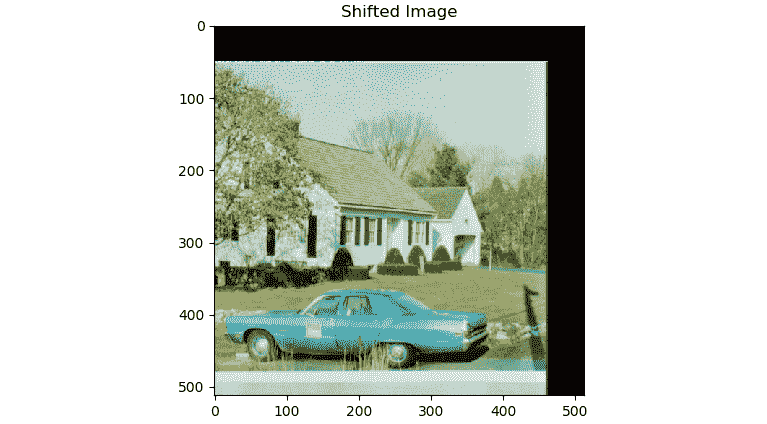import cv2
import matplotlib.pyplot as plt
input = cv2.cvtColor(img, cv2.COLOR_BGR2RGB)
rows, cols, channel = img.shape
R = cv2.getRotationMatrix2D((cols/2, rows/2), 45, 0.5)
output = cv2.warpAffine(input, R, (cols, rows))
plt.imshow(output)
plt.title('Rotated and Downscaled Image')
plt.show()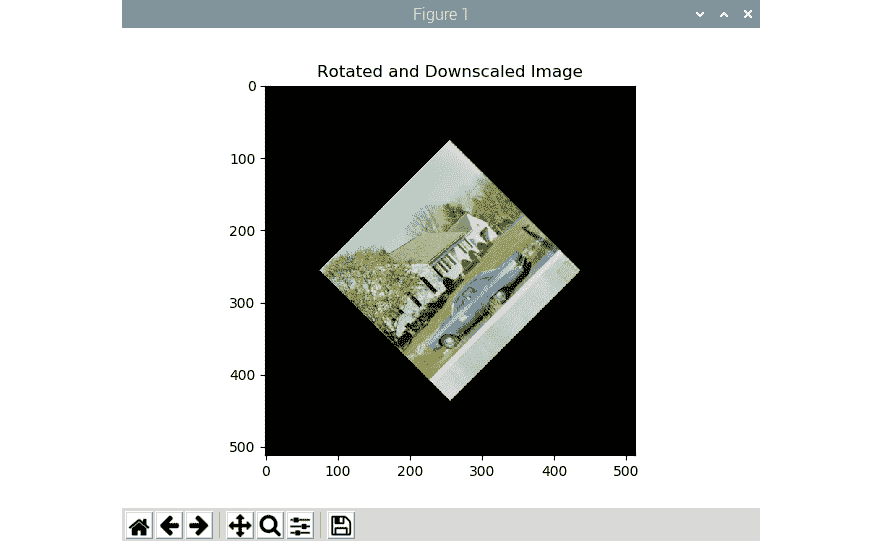import cv2
from time import sleep
rows, cols, channels = image.shape
angle = 0
while(1):
if angle == 360:
angle = 0
M = cv2.getRotationMatrix2D((cols/2, rows/2), angle, 1)
rotated = cv2.warpAffine(image, M, (cols, rows))
cv2.imshow('Rotating Image', rotated)
angle = angle +1
sleep(0.2)
if cv2.waitKey(1) == 27 :
break
cv2.destroyAllWindows()


import cv2
from time import sleep
cap = cv2.VideoCapture(0)
rows, cols, channels = frame.shape
angle = 0
while(1):
if angle == 360:
angle = 0
M = cv2.getRotationMatrix2D((cols/2, rows/2), angle, 1)
rotated = cv2.warpAffine(frame, M, (cols, rows))
cv2.imshow('Rotating Image', rotated)
angle = angle +1
sleep(0.2)
if cv2.waitKey(1) == 27 :
break
cv2.destroyAllWindows()


import cv2
import numpy as np
from matplotlib import pyplot as plt
input = cv2.cvtColor(image, cv2.COLOR_BGR2RGB )
rows, cols, channels = input.shape
points1 = np.float32([[100, 100], [300, 100], [100, 300]])
points2 = np.float32([[200, 150], [400, 150], [100, 300]])
A = cv2.getAffineTransform(points1, points2)
output = cv2.warpAffine(input, A, (cols, rows))
plt.subplot(121)
plt.imshow(input)
plt.title('Input')
plt.subplot(122)
plt.imshow(output)
plt.title('Affine Output')
plt.show()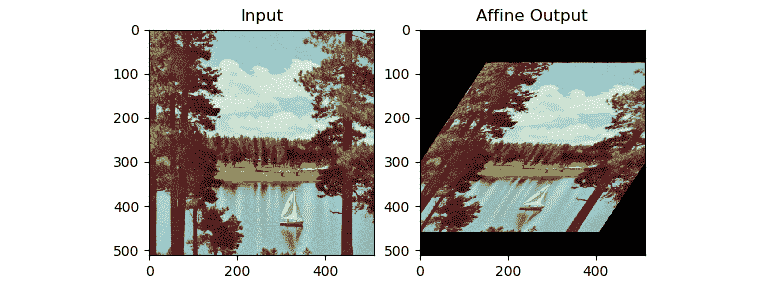# 图像的透视变换

import cv2
import numpy as np
from matplotlib import pyplot as plt
input = cv2.cvtColor(image, cv2.COLOR_BGR2RGB )
rows, cols, channels = input.shape
points1 = np.float32([[0, 0], [400, 0], [0, 400], [400, 400]])
points2 = np.float32([[0,0], [300, 0], [0, 300], [300, 300]])
P = cv2.getPerspectiveTransform(points1, points2)
output = cv2.warpPerspective(input, P, (300, 300))
plt.subplot(121)
plt.imshow(input)
plt.title('Input Image')
plt.subplot(122)
plt.imshow(output)
plt.title('Perspective Transform')
plt.show()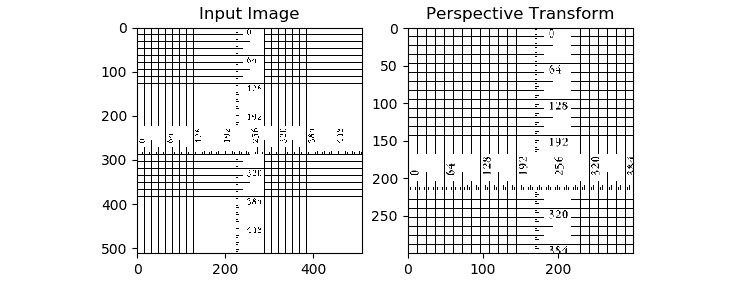# 对图像应用阈值

OpenCV cv2.threshold()函数将阈值应用于图像。 它接受图像，阈值，最大值和阈值处理技术作为参​​数，并返回阈值图像作为输出。 如果最大值大于阈值，则此函数将最大值分配给像素。 正如我们前面提到的，此方法存在多种变化。 让我们详细了解所有阈值技术。

• cv2.THRESH_BINARY：如果强度（xy）>脱粒，则设置强度(x, y) = maxVal； 否则，设置强度(x, y) = 0
• cv2.THRESH_BINARY_INV：如果强度（xy）>脱粒，则设置强度(x, y) = 0; 否则，设置强度(x, y) = maxVal
• cv2.THRESH_TRUNC：如果强度（xy）>脱粒，然后设置强度（xy）=阈值； 否则保持强度(x, y)不变。
• cv2.THRESH_TOZERO：如果强度（xy）>脱粒； 然后保持强度(x, y)不变； 否则，设置强度(x, y) = 0
• cv2.THRESH_TOZERO_INV：如果强度（xy）>脱粒，则设置强度(x, y) = 0; 否则，保持强度(x, y)不变。

import cv2
import matplotlib.pyplot as plt
import numpy as np
th = 127
max_val = 255
ret, o1 = cv2.threshold(img, th, max_val,
cv2.THRESH_BINARY)
print(o1)
ret, o2 = cv2.threshold(img, th, max_val,
cv2.THRESH_BINARY_INV)
ret, o3 = cv2.threshold(img, th, max_val,
cv2.THRESH_TOZERO)
ret, o4 = cv2.threshold(img, th, max_val,
cv2.THRESH_TOZERO_INV)
ret, o5 = cv2.threshold(img, th, max_val,
cv2.THRESH_TRUNC)
titles = ['Input Image', 'BINARY', 'BINARY_INV',
'TOZERO', 'TOZERO_INV', 'TRUNC']
output = [img, o1, o2, o3, o4, o5]
for i in range(6):
plt.subplot(2, 3, i+1)
plt.imshow(output[i], cmap='gray')
plt.title(titles[i])
plt.axis('off')
plt.show()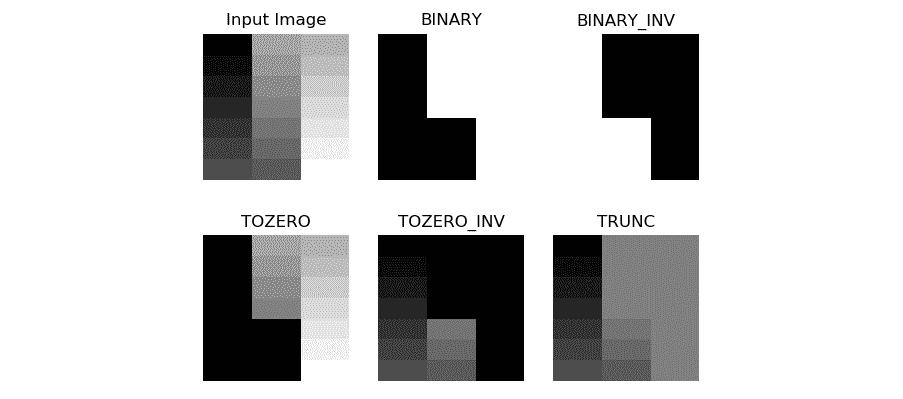import RPi.GPIO as GPIO
import cv2
thresh = 127
cap = cv2.VideoCapture(0)
GPIO.setmode(GPIO.BOARD)
GPIO.setwarnings(False)
button1 = 7
button2 = 11
GPIO.setup(button1, GPIO.IN, GPIO.PUD_UP)
GPIO.setup(button2, GPIO.IN, GPIO.PUD_UP)
while True:
button1_state = GPIO.input(button1)
if button1_state == GPIO.LOW and thresh < 256:
thresh = thresh + 1
button2_state = GPIO.input(button2)
if button2_state == GPIO.LOW and thresh > -1:
thresh = thresh - 1
ret1, output = cv2.threshold(frame, thresh, 255,
cv2.THRESH_BINARY)
print(thresh)
cv2.imshow('Thresholding App', output)
if cv2.waitKey(1) == 27:
break
cv2.destroyAllWindows()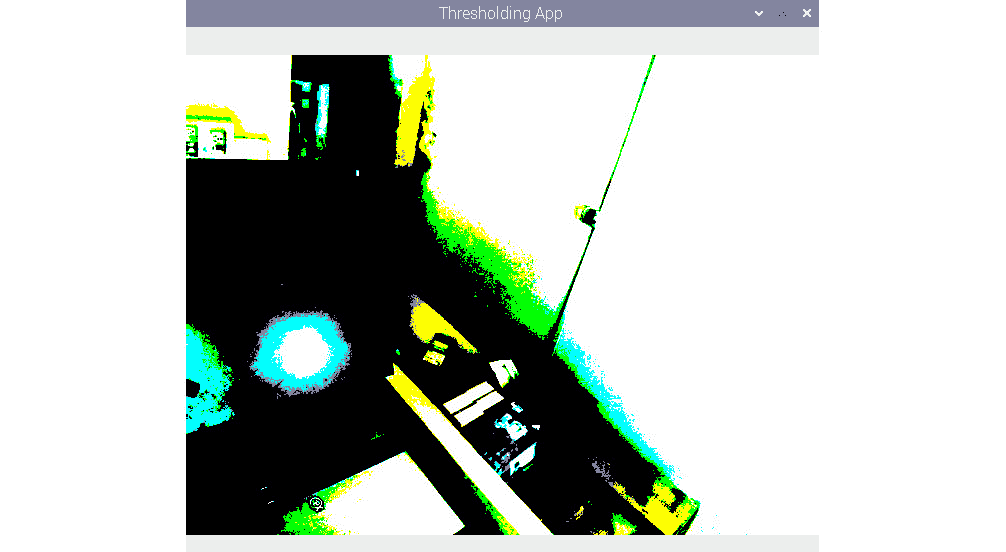## 大津的二值化方法

ret, output = cv2.threshold(image, 0, 255, cv2.THRESH_BINARY + cv2.THRESH_OTSU )


## 自适应阈值

cv2.adaptiveThreshold()方法接受源图像，最大值，自适应阈值方法，阈值算法，块大小和常数作为输入，并生成阈值图像作为输出。 下面显示了如何使用均值和高斯方法确定邻域以确定阈值值：

import cv2
import matplotlib.pyplot as plt
block_size = 123
constant = 6
cv2.THRESH_BINARY,
block_size, constant)
cv2.THRESH_BINARY,
block_size, constant)
output = [img, th1, th2]
for i in range(3):
plt.subplot(1, 3, i+1)
plt.imshow(output[i], cmap='gray')
plt.title(titles[i])
plt.xticks([])
plt.yticks([])
plt.show()